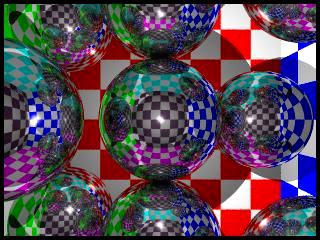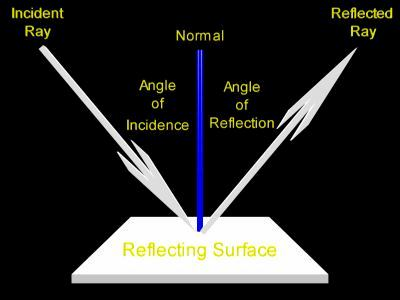# Ray Optics, Light ReflectionIn the ray traced image to the left several polished chrome spheres reflect light from varied positions within a checkerboard tiled room. This picture shows multiple light reflections for curved surfaces. At first we will look at a single ray of light reflecting off of a flat surface, such as a common mirror.

Below a light beam reflects at the surface of a flat mirror. This diagram demonstrates the reflective behavior of any single ray of light. Explanations follow.

Click on the picture to change the origin of the light ray. Drag slowly.

In the above diagram the reflecting surface is drawn in green, the normal is drawn in blue.Here's a simple reflection, one that happens on a flat surface. To the left is a picture of such a situation. Here a single ray of light strikes a surface and is reflected from it.

Descriptions for the terms in this diagram:

• The ray of light which strikes the surface is called the incident ray.
• The ray of light which leaves the surface is called the reflected ray.
• A line perpendicular to the surface is imagined at the point of reflection. This line is called a normal. In this context the word normal means perpendicular. In the above diagram the normal is colored blue.
• The angle between the incident ray and the normal is called the angle of incidence, or the incident angle.
• The angle between the reflected ray and the normal is called the angle of reflection, or the reflected angle.

Notice that the angle of incidence is equal to the angle of reflection.

The reflection of light is often discussed using phrases such as "a ray of light bounces off of a mirror." This is because when a light ray reflects at the surface of a mirror it follows a path similar in behavior to a pool ball bouncing off of a cushion on a pool table. However, unusual it may sound at first, it is not really the best idea to describe the reflection of a light ray using words like 'bounce'.  Light rays, though, can be considered to have a wave nature. Waves are often described as turning back, not bouncing, when they reflect.

Custom Search